# Intro to Digital Signal Processing by Robert Nowak, et al - HTML preview

PLEASE NOTE: This is an HTML preview only and some elements such as links or page numbers may be incorrect.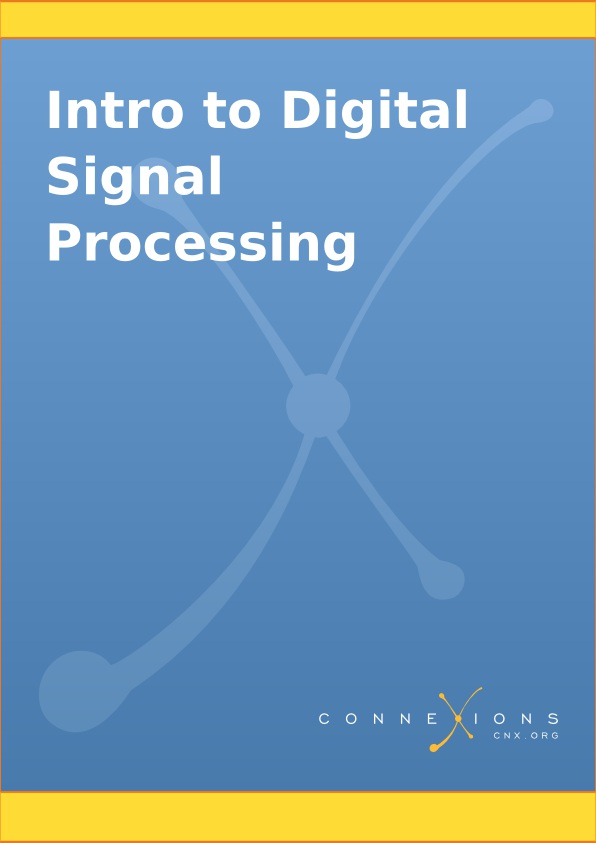Intro to Digital Signal Processing

Collection edited by: Robert Nowak

Content authors: Robert Nowak, Don Johnson, Michael Haag, Behnaam Aazhang, Nick

Kingsbury, Benjamin Fite, Melissa Selik, Richard Baraniuk, Dan Calderon, Ivan Selesnick, Bill

Wilson, Hyeokho Choi, Douglas Jones, Swaroop Appadwedula, Matthew Berry, Mark Haun, Dima

Moussa, Daniel Sachs, Roy Ha, Justin Romberg, and Phil Schniter

Online: < http://cnx.org/content/col10203/1.4>

This selection and arrangement of content as a collection is copyrighted by Robert Nowak.

Collection structure revised: 2004/01/22

For copyright and attribution information for the modules contained in this collection, see the " Attributions" section at the end of the collection.

Intro to Digital Signal Processing

Chapter 1. DSP Systems I

1.1. Image Restoration Basics

Image Restoration

Frequency Domain Analysis

1.2. Digital Image Processing Basics

Digital Image Processing

1.3. 2D DFT

2D DFT

Inverse 2D DFT

2D DFT and Convolution

Circular Convolution

Computing the 2D DFT

1.4. Images: 2D signals

Image Processing

Linear Shift Invariant Systems

2D Fourier Analysis

2D Sampling Theory

Nyquist Theorem

1.5. DFT as a Matrix Operation

Matrix Review

Representing DFT as Matrix Operation

1.6. Fast Convolution Using the FFT

Important Application of the FFT

Summary of DFT

What is the DFT

Inverse DFT (IDFT)

Circular Convolution

Regular Convolution from Circular Convolution

The Fast Fourier Transform (FFT)

Fast Convolution

1.7. The FFT Algorithm

The Fast Fourier Transform FFT

How does the FFT work?

Decimation in Time FFT

Derivation

1.8. The DFT: Frequency Domain with a Computer Analysis

Introduction

Sampling DTFT

Choosing M

Case 1

Case 2

Discrete Fourier Transform (DFT)

Interpretation

Remark 1

Remark 2

Periodicity of the DFT

A Sampling Perspective

Inverse DTFT of S(ω)

Connections

1.9. Discrete-Time Processing of CT Signals

DT Processing of CT Signals

Analysis

Summary

Note

Application: 60Hz Noise Removal

DSP Solution

Sampling Period/Rate

Digital Filter

1.10. Sampling CT Signals: A Frequency Domain Perspective

Understanding Sampling in the Frequency Domain

Sampling

Relating x[n] to sampled x(t)

1.11. Filtering with the DFT

Introduction

Compute IDFT of Y[k]

DFT Pair

Regular Convolution from Periodic Convolution

DSP System

1.12. Ideal Reconstruction of Sampled Signals

Reconstruction of Sampled Signals

Step 1

Step 2

Ideal Reconstruction System

1.13. Amplitude Quantization

1.14. Classic Fourier Series

Chapter 2. Random Signals

2.1. Introduction to Random Signals and Processes

Signals: Deterministic vs. Stochastic

Deterministic Signals

Stochastic Signals

Random Process

2.2. Introduction to Stochastic Processes

Definitions, distributions, and stationarity

2.3. Random Signals

Example - Detection of a binary signal in noise

2.4. Stationary and Nonstationary Random Processes

Introduction

Distribution and Density Functions

Stationarity

First-Order Stationary Process

Second-Order and Strict-Sense Stationary Process

Wide-Sense Stationary Process

2.5. Random Processes: Mean and Variance

Mean Value

Properties of the Mean

Mean-Square Value

Variance

Standard Deviation

Properties of Variance

Time Averages

Estimating the Mean

Estimating the Variance

Example

2.6. Correlation and Covariance of a Random Signal

Covariance

Useful Properties

Correlation

Correlation Coefficient

Example

Matlab Code for Example

2.7. Autocorrelation of Random Processes

Autocorrelation Function

Properties of Autocorrelation

Estimating the Autocorrleation with Time-Averaging

Examples

2.8. Crosscorrelation of Random Processes

Crosscorrelation Function

Properties of Crosscorrelation

Examples

Chapter 3. Filter Design I (Z-Transform)

3.1. Difference Equation

Introduction

General Formulas for the Difference Equation

Difference Equation

Conversion to Z-Transform

Conversion to Frequency Response

Example

Solving a LCCDE

Direct Method

Homogeneous Solution

Particular Solution

Indirect Method

3.2. The Z Transform: Definition

Basic Definition of the Z-Transform

The Complex Plane

Region of Convergence

3.3. Table of Common z-Transforms

3.4. Poles and Zeros

Introduction

Pole/Zero Plots

Repeated Poles and Zeros

Pole-Zero Cancellation

3.5. Rational Functions and the Z-Transform

Introduction

Properties of Rational Functions

Roots

Discontinuities

Domain

Intercepts

Rational Functions and the Z-Transform

Conclusion

3.6. The Complex Plane

Complex Plane

Rectangular Coordinates

Polar Form

3.7. Region of Convergence for the Z-transform

Introduction

The Region of Convergence

Properties of the Region of Convergencec

Examples

Graphical Understanding of ROC

Conclusion

3.8. Understanding Pole/Zero Plots on the Z-Plane

Introduction to Poles and Zeros of the Z-Transform

The Z-Plane

Examples of Pole/Zero Plots

Interactive Demonstration of Poles and Zeros

Applications for pole-zero plots

Stability and Control theory

Pole/Zero Plots and the Region of Convergence

Frequency Response and Pole/Zero Plots

3.9. Zero Locations of Linear-Phase FIR Filters

Zero Locations of Linear-Phase Filters

ZEROS LOCATIONS

ZERO LOCATIONS: AUTOMATIC ZEROS

ZERO LOCATIONS: EXAMPLES

3.10. Discrete Time Filter Design

Estimating Frequency Response from Z-Plane

Drawing Frequency Response from Pole/Zero Plot

Interactive Filter Design Illustration

Conclusion

Chapter 4. Filter Design II

4.1. Bilinear Transform

Chapter 5. Filter Design III

5.1. Linear-Phase FIR Filters

THE AMPLITUDE RESPONSE

WHY LINEAR-PHASE?

WHY LINEAR-PHASE: EXAMPLE

WHY LINEAR-PHASE: EXAMPLE (2)

WHY LINEAR-PHASE: MORE

5.2. Four Types of Linear-Phase FIR Filters

FOUR TYPES OF LINEAR-PHASE FIR FILTERS

TYPE I: ODD-LENGTH SYMMETRIC

TYPE II: EVEN-LENGTH SYMMETRIC

TYPE III: ODD-LENGTH ANTI-SYMMETRIC

TYPE IV: EVEN-LENGTH ANTI-SYMMETRIC

5.3. Design of Linear-Phase FIR Filters by DFT-Based Interpolation

DESIGN OF FIR FILTERS BY DFT-BASED INTERPOLATION

Types I and II

Types III and IV

EXAMPLE: DFT-INTERPOLATION (TYPE I)

SUMMARY: IMPULSE AND AMP RESPONSE

5.4. Design of Linear-Phase FIR Filters by General Interpolation

DESIGN OF FIR FILTERS BY GENERAL INTERPOLATION

EXAMPLE

LINEAR-PHASE FIR FILTERS: PROS AND CONS

5.5. Linear-Phase FIR Filters: Amplitude Formulas

SUMMARY: AMPLITUDE FORMULAS

AMPLITUDE RESPONSE CHARACTERISTICS

EVALUATING THE AMPLITUDE RESPONSE

Types I and II

Types III and IV

Modules for Further Study

5.6. FIR Filter Design using MATLAB

FIR Filter Design Using MATLAB

Design by windowing

Parks-McClellan FIR filter design

5.7. MATLAB FIR Filter Design Exercise

FIR Filter Design MATLAB Exercise

Design by windowing

Parks-McClellan Optimal Design

5.8. Parks-McClellan Optimal FIR Filter Design

Chapter 6. Wiener Filter Design

Introduction

MATLAB Simulation

Processor Implementation

Extensions

References

Chapter 8. Wavelets and Filterbanks

8.1. Haar Wavelet Basis

Introduction

Basis Comparisons

Haar Wavelet Transform

Haar Wavelet Demonstration

8.2. Orthonormal Wavelet Basis

8.3. Continuous Wavelet Transform

8.4. Discrete Wavelet Transform: Main Concepts

Main Concepts

8.5. The Haar System as an Example of DWT

Index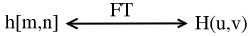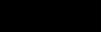Chapter 1. DSP Systems I

1.1. Image Restoration Basics*

Image Restoration

In many applications ( e.g. , satellite imaging, medical imaging, astronomical imaging, poor-

quality family portraits) the imaging system introduces a slight distortion. Often images are

slightly blurred and image restoration aims at deblurring the image.

The blurring can usually be modeled as an LSI system with a given PSF h[ m, n] .

Figure 1.1.

Fourier Transform (FT) relationship between the two functions.

The observed image is

()

g[ m, n]= h[ m, n]* f[ m, n]

()

G( u, v)= H( u, v) F( u, v)

()

Example 1.1. Image Blurring

Above we showed the equations for representing the common model for blurring an image. In

Figure 1.2 we have an original image and a PSF function that we wish to apply to the image in order to model a basic blurred image.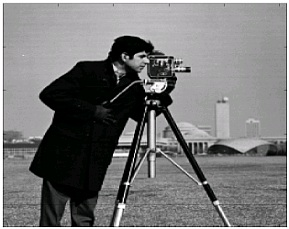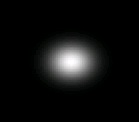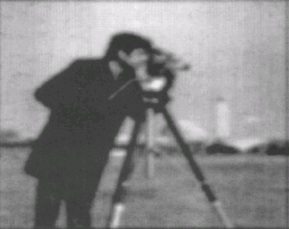(b)

(a)

Figure 1.2.

Once we apply the PSF to the original image, we receive our blurred image that is shown in

Figure 1.3:

Figure 1.3.

Frequency Domain Analysis

Example 1.1 looks at the original images in its typical form; however, it is often useful to look at our images and PSF in the frequency domain. In Figure 1.4, we take another look at the image blurring example above and look at how the images and results would appear in the frequency

domain if we applied the fourier transforms.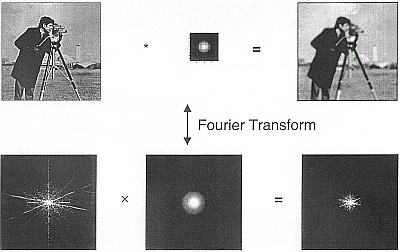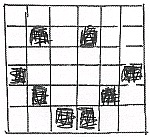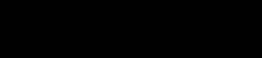Figure 1.4.

1.2. Digital Image Processing Basics*

Digital Image Processing

A sampled image gives us our usual 2D array of pixels f[ m, n] (Figure 1.5): Figure 1.5.

We illustrate a "pixelized" smiley face.

We can filter f[ m, n] by applying a 2D discrete-space convolution as shown below (where h[ m, n] is our PSF):

()

Example 1.2. Sampled Image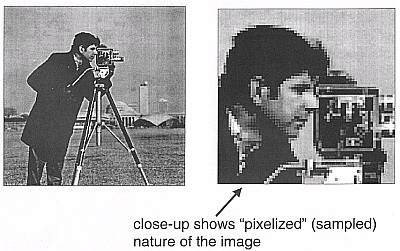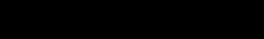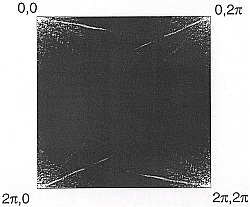Figure 1.6.

Illustrate the "pixelized" nature of all digital images.

We also have discrete-space FTS:

()

where F[ u, v] is analogous to DTFT in 1D.

"Convolution in Time" is the same as "Multiplication in Frequency"

()

g[ m, n]= h[ m, n]* f[ m, n]

which, as stated above, is the same as:

()

G[ u, v]= H[ u, v] F[ u, v]

Example 1.3. Magnitude of FT of Cameraman Image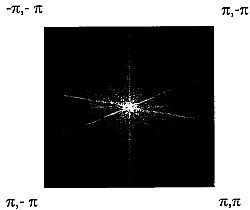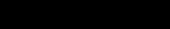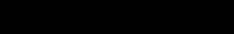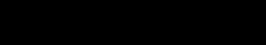Figure 1.7.

To get a better image, we can use the fftshift command in Matlab to center the Fourier

Transform. The resulting image is shown in Figure 1.8:

Figure 1.8.

1.3. 2D DFT*

2D DFT

To perform image restoration (and many other useful image processing algorithms) in a computer,

we need a Fourier Transform (FT) that is discrete and two-dimensional.

()

for k={0, , N−1} and l={0, , N−1} .

()

()

where the above equation (Equation) has finite support for an N x N image.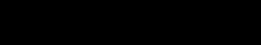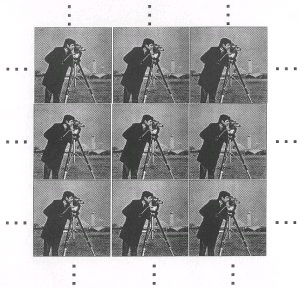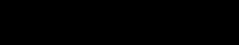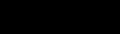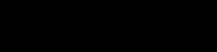Inverse 2D DFT

As with our regular fourier transforms, the 2D DFT also has an inverse transform that allows us to

reconstruct an image as a weighted combination of complex sinusoidal basis functions.

()

Example 1.4. Periodic Extensions

Figure 1.9.

Illustrate the periodic extension of images.

2D DFT and Convolution

The regular 2D convolution equation is

()

Example 1.5.

Below we go through the steps of convolving two two-dimensional arrays. You can think of f

as r